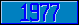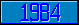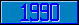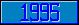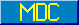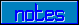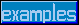## \$%TAN^MATH

### M[UMPS] by Example

Approved for inclusion in a future M[UMPS] language standard as part of the library for Trigonometric Mathematics.

This function returns the tangent of the value of its parameter (in radians).

Reference Value \$%TAN^MATH(–2) 2.185039863... \$%TAN^MATH(–1) –1.557407725... \$%TAN^MATH(0) 0 \$%TAN^MATH(1) 1.557407725... \$%TAN^MATH(2) –2.185039863...

The MDC has approved code to approximate the return value of this function. Implementors are encouraged to provide more accurate and efficient code).